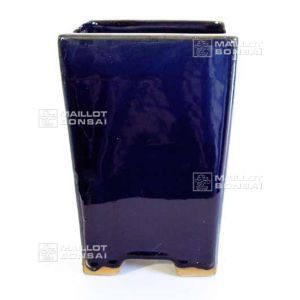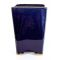##### The Japanese Bonsai specialist
Direct order Contact Help / Services Newsletter# 29 coloured glazed pot navy

› Bonsai pots › Cascade potsref. : 11474

18,00

Available quantity : 35Order

###### Description

Exterior: 10*10*14 cm. Interior: 8*8*12 cm. Colour:  marine blue. Our small, tall pots come in a range of vibrant shades to add a colour pop to your home and garden. Line them up on your windowsill, create a mini-pot arrangement of sedums or cacti, or else plant a cascading 'kengai' bonsai or semi-cascade 'han kengai' bonsai.

#cascade 3.7 #pots 3.4 #coloured 2.8 #kengai 2.6 #glazed 2.6 #bonsai 2.6 #colour 2.6 #navy 2.4 #your 2.4 #arrangement 2.1

###### Technical description
 shape color marine blue origin China Yixing

Formule
(( ROUND((CHAR_LENGTH(b.article_nom)-CHAR_LENGTH(REPLACE(b.article_nom, 'coloured', '')))/LENGTH('coloured')) + ROUND((CHAR_LENGTH(b.article_description)-CHAR_LENGTH(REPLACE(b.article_description, 'coloured', '')))/LENGTH('coloured')) ) * 2.8) + (( ROUND((CHAR_LENGTH(b.article_nom)-CHAR_LENGTH(REPLACE(b.article_nom, 'kengai', '')))/LENGTH('kengai')) + ROUND((CHAR_LENGTH(b.article_description)-CHAR_LENGTH(REPLACE(b.article_description, 'kengai', '')))/LENGTH('kengai')) ) * 2.6) + (( ROUND((CHAR_LENGTH(b.article_nom)-CHAR_LENGTH(REPLACE(b.article_nom, 'colour', '')))/LENGTH('colour')) + ROUND((CHAR_LENGTH(b.article_description)-CHAR_LENGTH(REPLACE(b.article_description, 'colour', '')))/LENGTH('colour')) ) * 2.6) + (( ROUND((CHAR_LENGTH(b.article_nom)-CHAR_LENGTH(REPLACE(b.article_nom, 'bonsai', '')))/LENGTH('bonsai')) + ROUND((CHAR_LENGTH(b.article_description)-CHAR_LENGTH(REPLACE(b.article_description, 'bonsai', '')))/LENGTH('bonsai')) ) * 2.6) + (( ROUND((CHAR_LENGTH(b.article_nom)-CHAR_LENGTH(REPLACE(b.article_nom, 'glazed', '')))/LENGTH('glazed')) + ROUND((CHAR_LENGTH(b.article_description)-CHAR_LENGTH(REPLACE(b.article_description, 'glazed', '')))/LENGTH('glazed')) ) * 2.6) + (( ROUND((CHAR_LENGTH(b.article_nom)-CHAR_LENGTH(REPLACE(b.article_nom, 'navy', '')))/LENGTH('navy')) + ROUND((CHAR_LENGTH(b.article_description)-CHAR_LENGTH(REPLACE(b.article_description, 'navy', '')))/LENGTH('navy')) ) * 2.4) + (( ROUND((CHAR_LENGTH(b.article_nom)-CHAR_LENGTH(REPLACE(b.article_nom, 'your', '')))/LENGTH('your')) + ROUND((CHAR_LENGTH(b.article_description)-CHAR_LENGTH(REPLACE(b.article_description, 'your', '')))/LENGTH('your')) ) * 2.4) + (( ROUND((CHAR_LENGTH(b.article_nom)-CHAR_LENGTH(REPLACE(b.article_nom, 'arrangement', '')))/LENGTH('arrangement')) + ROUND((CHAR_LENGTH(b.article_description)-CHAR_LENGTH(REPLACE(b.article_description, 'arrangement', '')))/LENGTH('arrangement')) ) * 2.1) + (( ROUND((CHAR_LENGTH(b.article_nom)-CHAR_LENGTH(REPLACE(b.article_nom, 'windowsill', '')))/LENGTH('windowsill')) + ROUND((CHAR_LENGTH(b.article_description)-CHAR_LENGTH(REPLACE(b.article_description, 'windowsill', '')))/LENGTH('windowsill')) ) * 2) + (( ROUND((CHAR_LENGTH(b.article_nom)-CHAR_LENGTH(REPLACE(b.article_nom, 'cascading', '')))/LENGTH('cascading')) + ROUND((CHAR_LENGTH(b.article_description)-CHAR_LENGTH(REPLACE(b.article_description, 'cascading', '')))/LENGTH('cascading')) ) * 1.9)

## Secure payment## Delivery

Our logistic partners :04 74 55 23 48
Pépinière MAILLOT-BONSAÏ
Le Bois Frazy
01990 RELEVANT - FRANCE
on appointment